﻿ 20-sim webhelp > Toolboxes > Frequency Domain Toolbox > Linear System Editor > Linear System Editor

# Linear System Editor

Navigation:  Toolboxes > Frequency Domain Toolbox > Linear System Editor >

# Linear System EditorThe Linear System Editor of 20-sim allows you to enter or edit linear time- invariant models. It supports continuous and discrete-time single input / single output (SISO) systems with and without time delay. You can open the Linear System Editor from the Tools menu of the 20-sim Editor or by clicking Go Down on the Linear System model. A linear system can also be the result of a linearization operation in the 20-sim Simulator.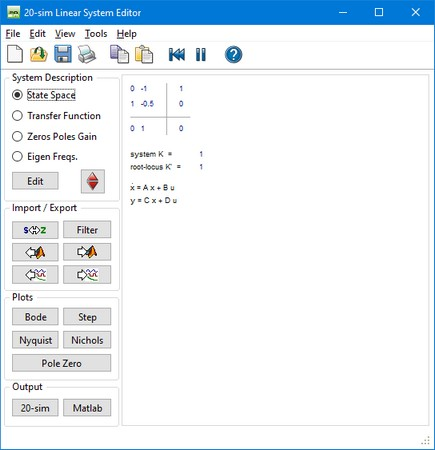Some menu items are of particular importance.

 • File: You can use the file menu to open, import and export linear systems.
 • Edit - Tolerance: You can specify the tolerance for transforming between state-space and transfer functions.
 • Edit - Reduce System: You can reduce the system order by pole-zero cancelation. The distance between poles and zeros to match for cancelation is given by the Reduction Tolerance.
 • View: the view menu can be used for holding, clearing and refreshing plots.

## System Description

 • Pressing the Edit button will open a dialog for editing the linear system. Each description (State Space, Transfer Function, Zeros Poles Gain or Eigen Frequency) has a special dialog and can be used to specify continuous-time and discrete-time systems.
 • In the 20-sim Simulator, out of an existing (non-linear) model a symbolic linear-system can be derived by means of linearization. This means that the relevant model parameters are preserved in the Linear System and the parameters button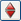can be used to change parameters.

## Import/Export

 • Pressing the s<->z  button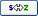will transform a continuous-time linear system into a discrete-time linear system and back.
 • Pressing the Filter button opens the Filter Editor, where a filter can be designed. This filter can then be combined with the current linear system or replace the current linear system. If the linear system is a discrete-time system, the designed analog filter is automatically transferred into its digital equivalent.
 • Pressing the From Matlab button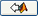and the To Matlab button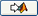allows for an instant exchange of the linear system with the Matlab workspace. Information is transferred numerically (no parameter relations are preserved)
 • The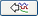button is only active when a Linear System has been imported through linearization. Clicking the button will import parameters from the simulation.
 • The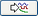button is only active when a Linear System has been imported through linearization. Clicking the button will export the current parameters to the simulation.

## Plots

You can inspect the time- and frequency responses of the linear system using:

 • Step Response
 • Bode Plot
 • Nyquist Plot
 • Nichols Chart
 • Poles and Zeros (including root locus)

## Output

 • Clicking the 20-sim button will export the linear system as a new 20-sim submodel.
 • Clicking the Matlab button will export the 20-sim Linear System to a Matlab m-file. If the Linear System is symbolic, all parameter relations are preserved.